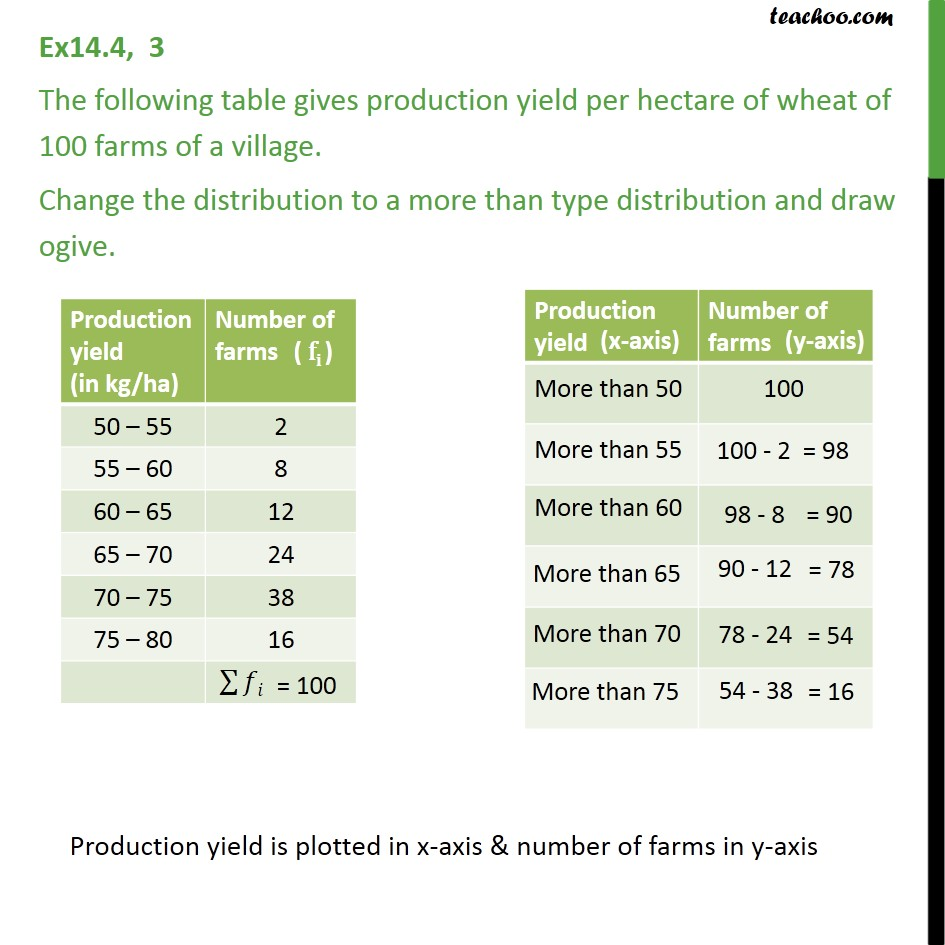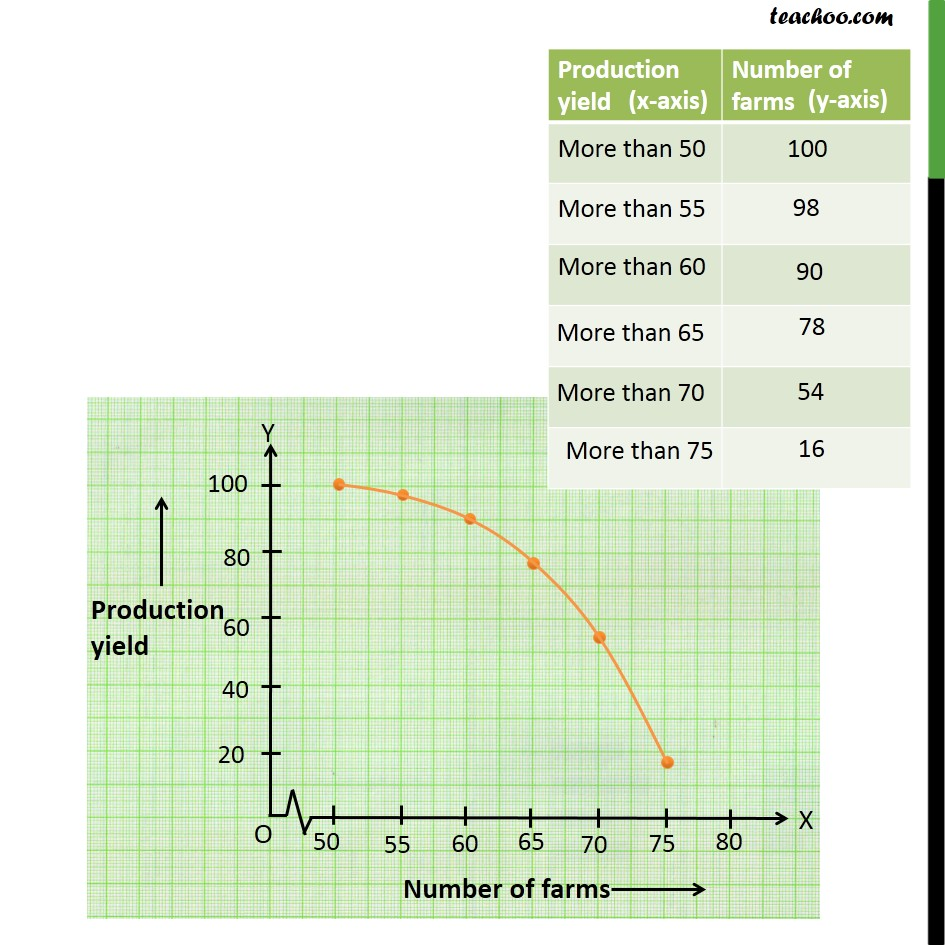1. Chapter 14 Class 10 Statistics
2. Concept wise
3. Less than , more than ogive

Transcript

Ex 14.4, 3 - Chapter 14 Class 10 The following table gives production yield per hectare of wheat of 100 farms of a village. Change the distribution to a more than type distribution and draw ogive. Production yield (in kg/ha) Number of farms 50 – 55 2 55 – 60 8 60 – 65 12 65 – 70 24 70 – 75 38 75 – 80 16 ∑▒𝑓𝑖 = 100 Production yield (x-axis) Number of farms(y-axis) More than 50 100 More than 55 100 - 2=98 More than 60 98 - 8=90 More than 65 90 - 12=78 More than 70 78 - 24=54 More than 75 54 - 38=16 Production yield is plotted in x-axis & number of farms in y-axis

Less than , more than ogive

Chapter 14 Class 10 Statistics
Concept wise